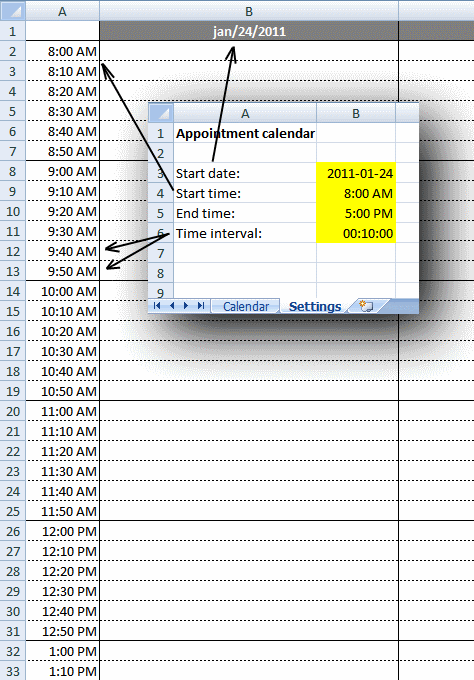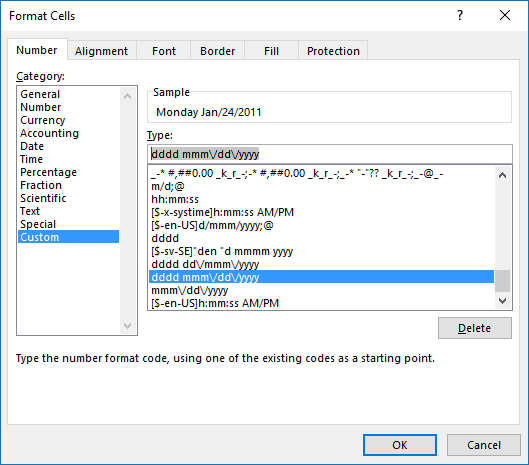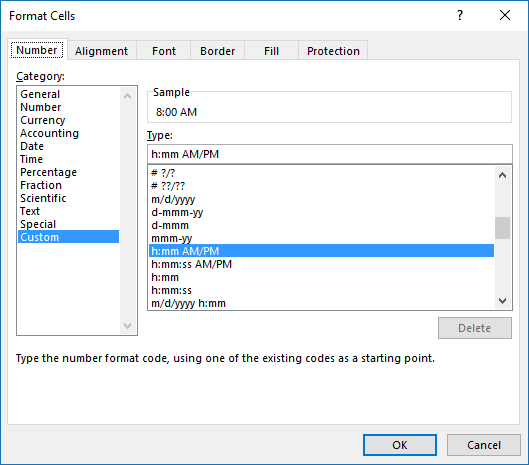Author: Oscar Cronquist Article last updated on January 10, 2019This weekly calendar is easy to customize, you can change calendar settings in sheet "Settings":

• Start date (preferably a Sunday or Monday)
• Start and end time
• Time interval

The calendar changes instantly based on the input values in sheet "Settings", then simply print the calendar.

Formula in cell B1:

=Settings!B3

This is a cell reference to sheet Settings and cell A3.

Formula in cell C1:

=B1+1

This returns the date in cell B1 and adds 1 meaning next day. Copy cell C1 and paste to cells to the right of cell C1.

Row 1 has the following cell formatting applied:Simply select cell C1 and press CTRL+ 1 to open the "Format Cells" dialog box, shown in the above picture. dddd returns the weekday, mmm returns month name abbreviated to three letters. dd returns the day of the date and yyyy returns the year.

Formula in cell A2:

=Settings!B4

This is a cell reference to sheet Settings and cell B4.

Formula in cell A3:

=IF(A2="","",IF((A2+Settings!\$B\$6)>Settings!\$B\$5,"",A2+Settings!\$B\$6))

Copy cell A3 and paste to cells below as far as needed.

### Explaining formula in cell A3

#### Step 1 - Add time value to interval value

A2+Settings!\$B\$6

becomes

0.333333333+0.00694444444444444

and returns 0.340277777777778

#### Step 2 - Check if value is larger than end time

The greater than sign is a logical operator that evaluates if the value is greater than end time value, we only want time values inside the range given in sheet settings cell B3 and B4.

(A2+Settings!\$B\$6)>Settings!\$B\$5

becomes

0.340277777777778>0.708333333333333

and returns FALSE.

#### Step 3 - Return nothing if TRUE and date + time value if FALSE

The IF function has three arguments, the first one must be a logical expression. If the expression evaluates to TRUE then one thing happens (argument 2) and if FALSE another thing happens (argument 3).

IF((A2+Settings!\$B\$6)>Settings!\$B\$5,"",A2+Settings!\$B\$6)

becomes

IF(FALSE,"",A2+Settings!\$B\$6)

becomes

IF(FALSE,"",0.340277777777778)

and returns 0.340277777777778.

#### Step 4 - If above cell is empty return nothing

IF(A2="", "", IF((A2+Settings!\$B\$6)>Settings!\$B\$5, "", A2+Settings!\$B\$6))

becomes

IF(A2="", "", 0.340277777777778)

becomes

IF(0.333333333333333="", "", 0.340277777777778)

becomes

IF(FALSE, "", 0.340277777777778)

and returns 0.340277777777778 formatted to 8:00 AM.

The image below shows the cell formatting applied to column A.### Get the Excel fileAppointment-calendar-template.xls